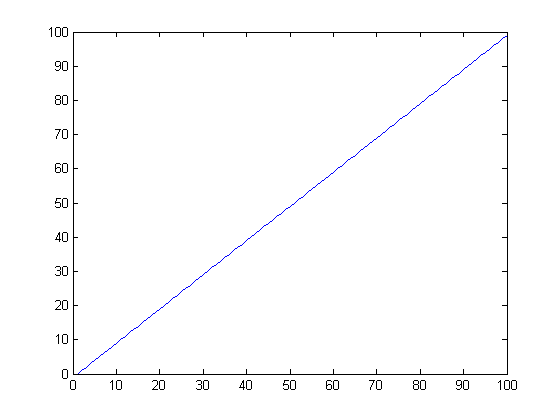# How To Send Data From The Arduino To MATLAB

by on July 4, 2011

in Arduino

I’ve been learning MATLAB lately and playing with the Arduino more and more, then I started to wonder how to make the Arduino communicate with this program, it turns out it’s not hard at all. See the code below.

## What The Program Will Do

It will send the value of a variable from the Arduino to MATLAB and plot them.

## Arduino Serial Code

If you have used the Serial class of the Arduino before this won’t be new to you. Simply print some data with the Serial.print() function.

```int i=0;
void setup()
{
Serial.begin(9600);
}

void loop()
{
Serial.println(i);
i++;
}
```

For those who haven’t used the serial class before all am doing in the code above is sending the value of the variable i at a baud rate of 9600.

## MATLAB Serial Class

This is just as easy the Arduino.

First we want to create an object of the class serial. Set the port to the port you are using to program the Arduino, am using COM4 to program it. Next set the baud rate you set in the Arduino code above.

```arduino=serial('COM4','BaudRate',9600);
```

Now we need to open the arduino as if it were a file.

```fopen(arduino)
```

We need to make a set of point for the x-axis of the plot

```x=linspace(1,100);
```

To read data from the Arduino we use the fscanf function, these will be our y-axis values on the plot. The %d means we are receiving a decimal number.

```for i=1:length(x)
y(i)=fscanf(arduino,'%d');
end
```

Now we close the Arduino communication and plot the data.

```fclose(arduino);
disp('making plot..')
plot(x,y);
```

## Putting All The MATLAB Code Together

This is the exact code I used.

```clear all
clc

arduino=serial('COM4','BaudRate',9600);

fopen(arduino);

x=linspace(1,100);

for i=1:length(x)
y(i)=fscanf(arduino,'%d');
end

fclose(arduino);
disp('making plot..')
plot(x,y);
```

## The Result

If everything worked you should get the following plot. Let us know how you are planning to use MATLAB and Arduino in the comments below.the plot generated by MATLAB using the variable i from the Arduino.

Previous post: